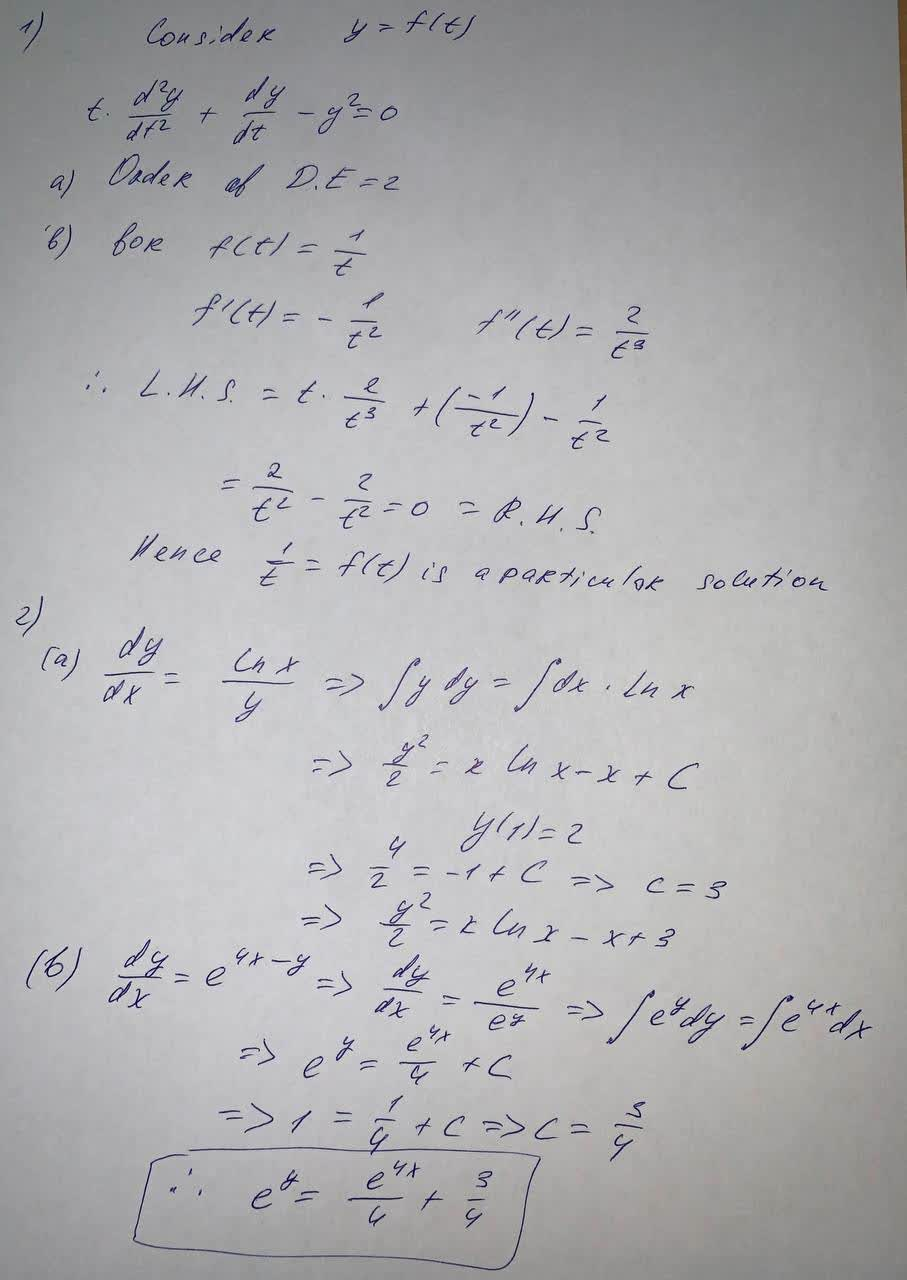#### Didn’t find what you are looking for?

Question# Consider the differential equation for a function f(t), tf"(t)+f'(t)-f((t))^2=0 a) What is the order of this differential equation? b) Show that f(t)=

Differential equations
ANSWEREDConsider the differential equation for a function f(t),
$$tf"(t)+f'(t)-f((t))^2=0$$
a) What is the order of this differential equation?
b) Show that $$f(t)=\frac{1}{t}$$ is a particular solution to this differential equation.
c) Find a particular solution with $$f(0)=0$$
2. Find the particular solutions to the differential equations with initial conditions:
a)$$\frac{dy}{dx}=\frac{\ln(x)}{y}$$ with $$y(1)=2$$
b)$$\frac{dy}{dx}=e^{4x-y}$$ with $$y(0)=0$$